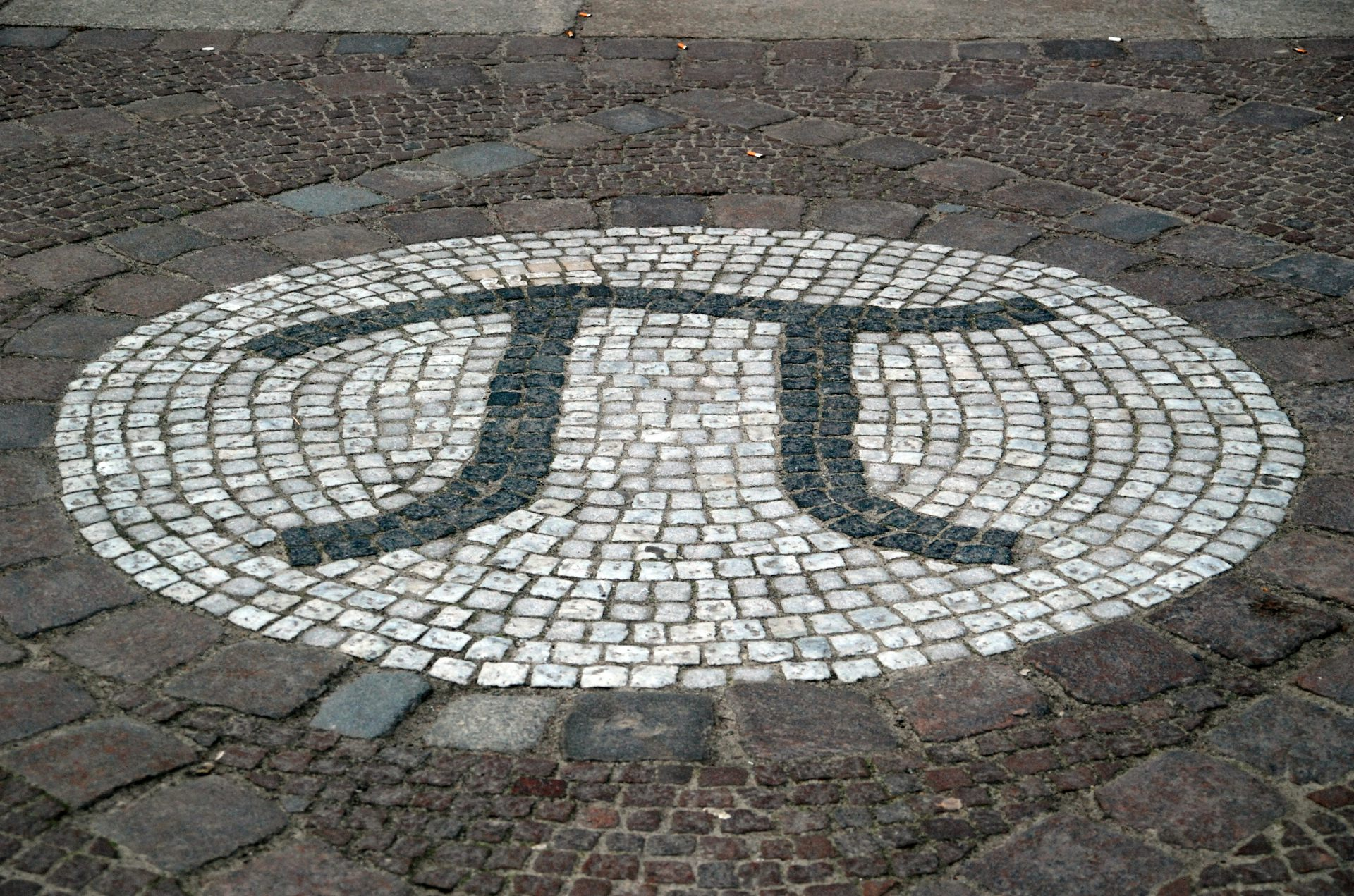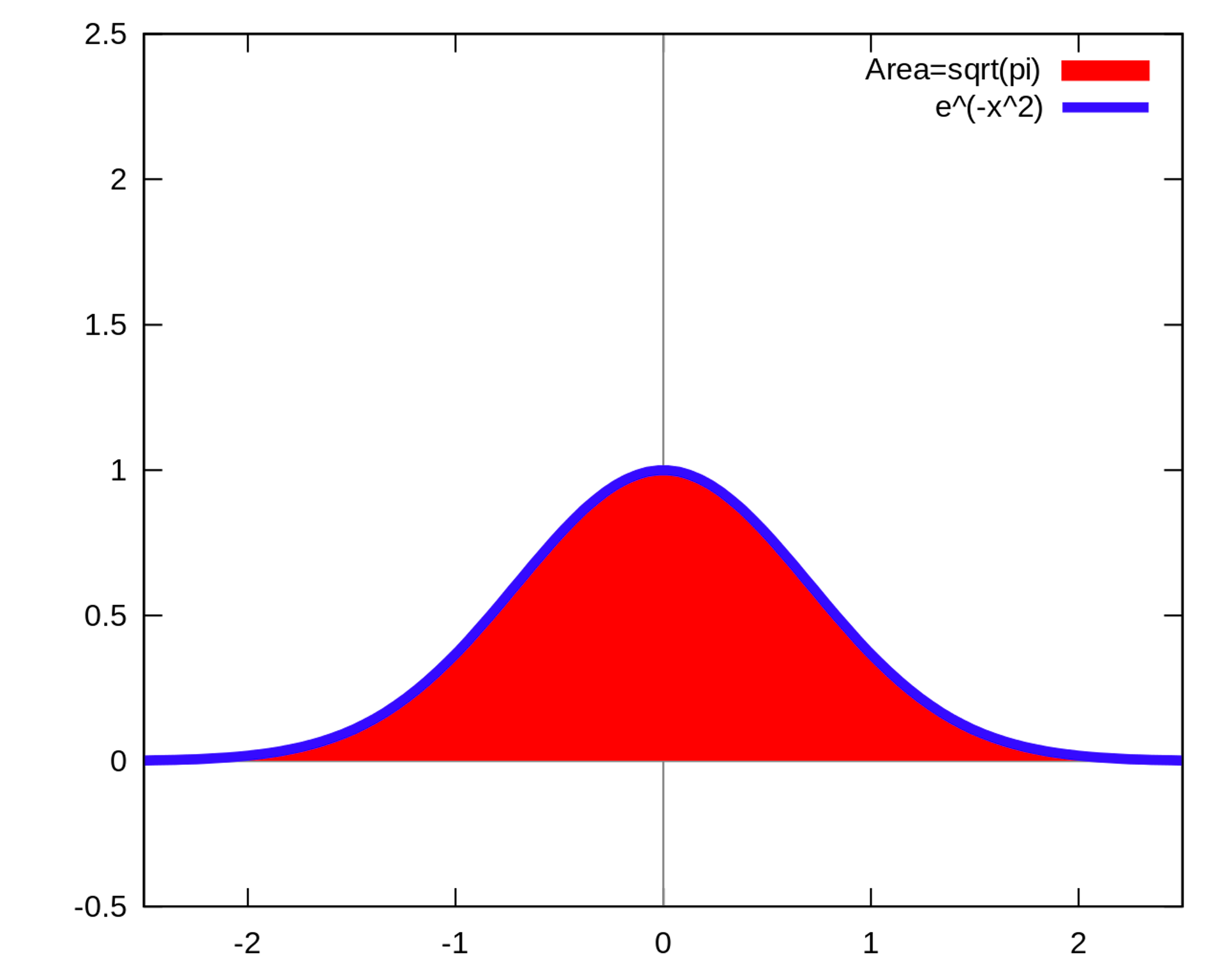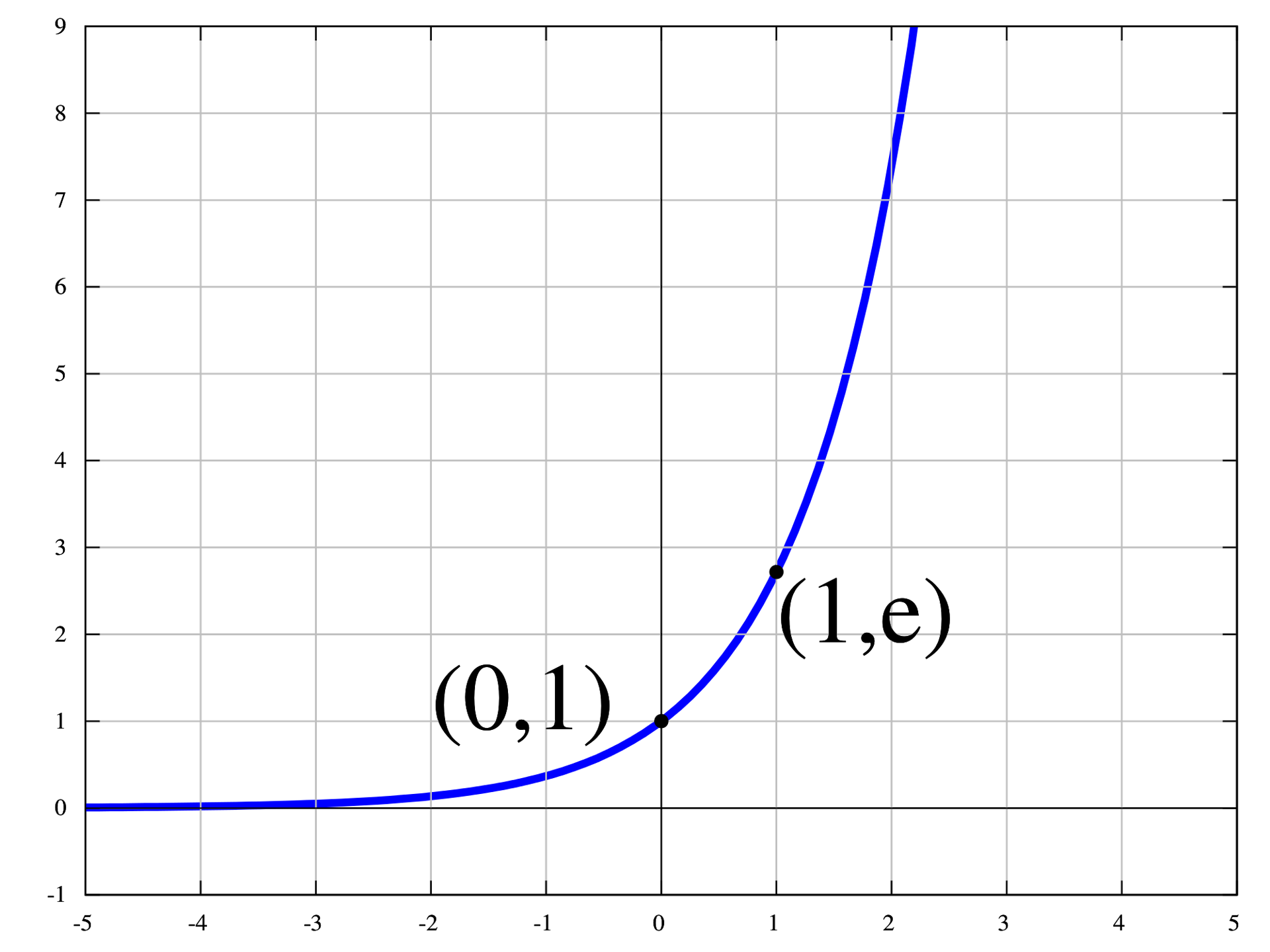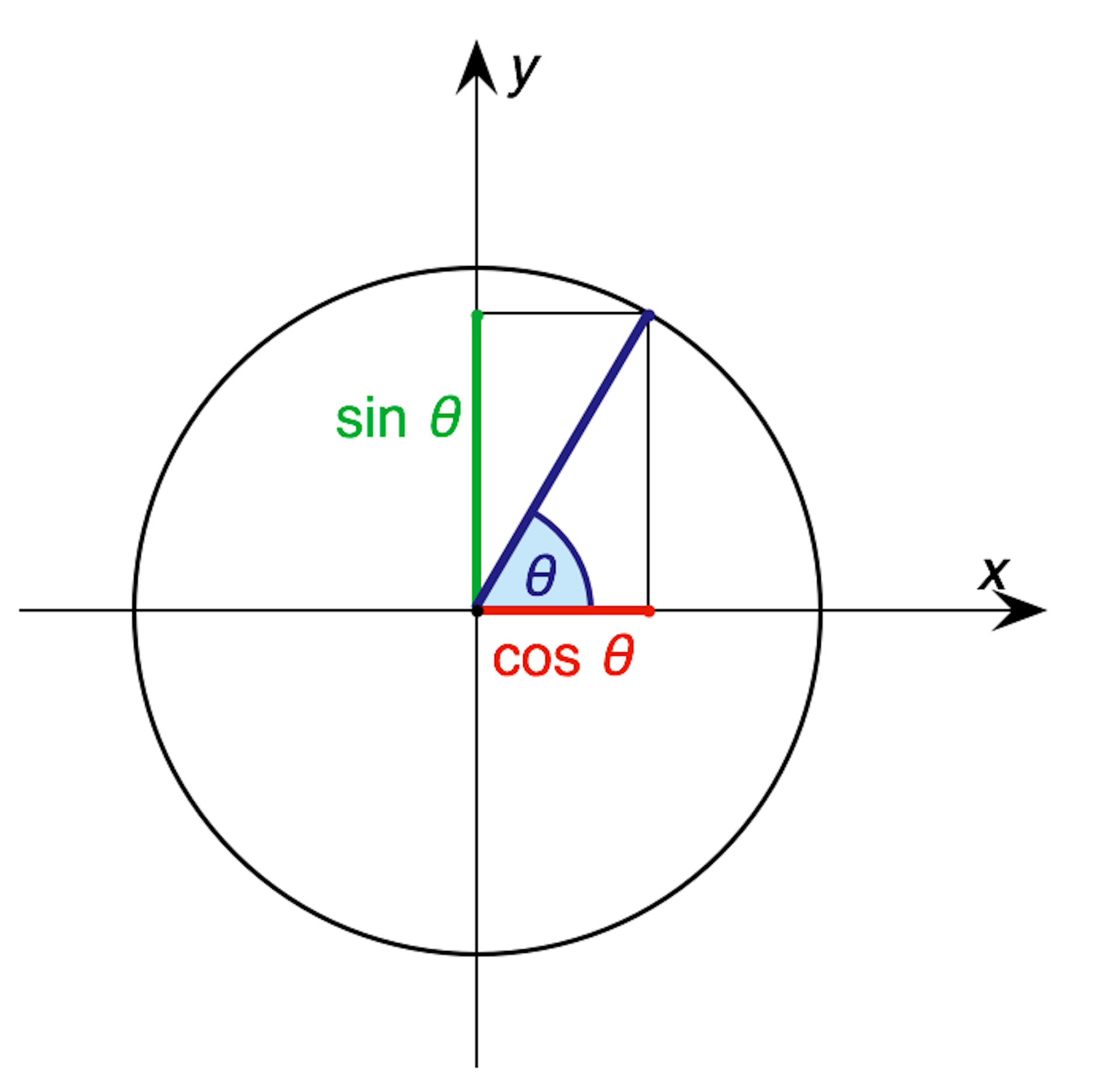Pi is at the center of all circles. Holger Motzkau, CC BY-SA

Happy Pi Day, where we celebrate the world’s most famous number. The exact value of π=3.14159… has fascinated people since ancient times, and mathematicians have computed trillions of digits. But why do we care? Would it actually matter if somebody got the 11,137,423,895,285th digit wrong?

Probably not. The world would keep on turning (with a circumference of 2πr). What matters about π isn’t so much the actual value as the idea, and the fact that π seems to crop up in lots of unexpected places.

Let’s start with the expected places. If a circle has radius r, then the circumference is 2πr. So if a circle has radius of one foot, and you walk around the circle in one-foot steps, then it will take you 2π = 6.28319… steps to go all the way around. Six steps isn’t nearly enough, and after seven you will have overshot. And since the value of π is irrational, no multiple of the circumference will be an even number of steps. No matter how many times you take a one-foot step, you’ll never come back exactly to your starting point.

From the circumference of a circle we get the area. Cut a pizza into an even number of slices, alternately colored yellow and blue. Lay all the blue slices pointing up, and all the yellow slices pointing down. Since each color accounts for half the circumference of the circle, the result is approximately a strip of height r and width πr, or area πr2. The more slices we have, the better the approximation is, so the exact area must be exactly πr2.

## Pi in other places

You don’t just get π in circular motion. You get π in any oscillation. When a mass bobs on a spring, or a pendulum swings back and forth, the position behaves just like one coordinate of a particle going around a circle.

If your maximum displacement is one meter and your maximum speed is one meter/second, it’s just like going around a circle of radius one meter at one meter/second, and your period of oscillation will be exactly 2π seconds.The area of the space under the normal-distribution curve is the square root of pi. Autopilot, CC BY-SA

Pi also crops up in probability. The function f(x)=e-x², where e=2.71828… is Euler’s number, describes the most common probability distribution seen in the real world, governing everything from SAT scores to locations of darts thrown at a target. The area under this curve is exactly the square root of π.

How did π get into it?! The two-dimensional function f(x)f(y) stays the same if you rotate the coordinate axes. Round things relate to circles, and circles involve π.

Another place we see π is in the calendar. A normal 365-day year is just over 10,000,000π seconds. Does that have something to do with the Earth going around the sun in a nearly circular orbit? Actually, no. It’s just coincidence, thanks to our arbitrarily dividing each day into 24 hours, each hour into 60 minutes, and each minute into 60 seconds.

What’s not coincidence is how the length of the day varies with the seasons. If you plot the hours of daylight as a function of the date, starting at next week’s equinox, you get the same sine curve that describes the position of a pendulum or one coordinate of circular motion.

More examples of π come up in calculus, especially in infinite series like
1 - (13) + (15) - (17) + (19) + ⋯ = π/4
and
12 + (12)2 + (13)2 + (14)2 + (15)2 + ⋯ = π2/6
(The first comes from the Taylor series of the arctangent of 1, and the second from the Fourier series of a sawtooth function.)

Also from calculus comes Euler’s mysterious equation
e + 1 = 0
relating the five most important numbers in mathematics: 0, 1, i, π, and e, where i is the (imaginary!) square root of -1.A graph of the exponential function y=e^x. Peter John Acklam, CC BY-SA

At first this looks like nonsense. How can you possibly take a number like e to an imaginary power?! Stay with me. The rate of change of the exponential function f(x)=ex is equal to the value of the function itself. To the left of the figure, where the function is small, it’s barely changing. To the right, where the function is big, it’s changing rapidly. Likewise, the rate of change of any function of the form f(x)=eax is proportional to eax.The relationship between an angle, its sine, cosine and a circle. 345Kai, CC BY-SA

We can then define f(x)= eix to be a complex function whose rate of change is i times the function itself, and whose value at 0 is 1. This turns out to be a combination of the trigonometric functions that describe circular motion, namely cos(x) + i sin(x). Since going a distance π takes you halfway around the unit circle, cos(π)=-1 and sin(π)=0, so e=-1.

Finally, some people prefer to work with τ=2π=6.28… instead of π. Since going a distance 2π takes you all the way around the circle, they would write that e = +1. If you find that confusing, take a few months to think about it. Then you can celebrate June 28 by baking two pies.

### Want to write?

Write an article and join a growing community of more than 95,100 academics and researchers from 3,098 institutions.